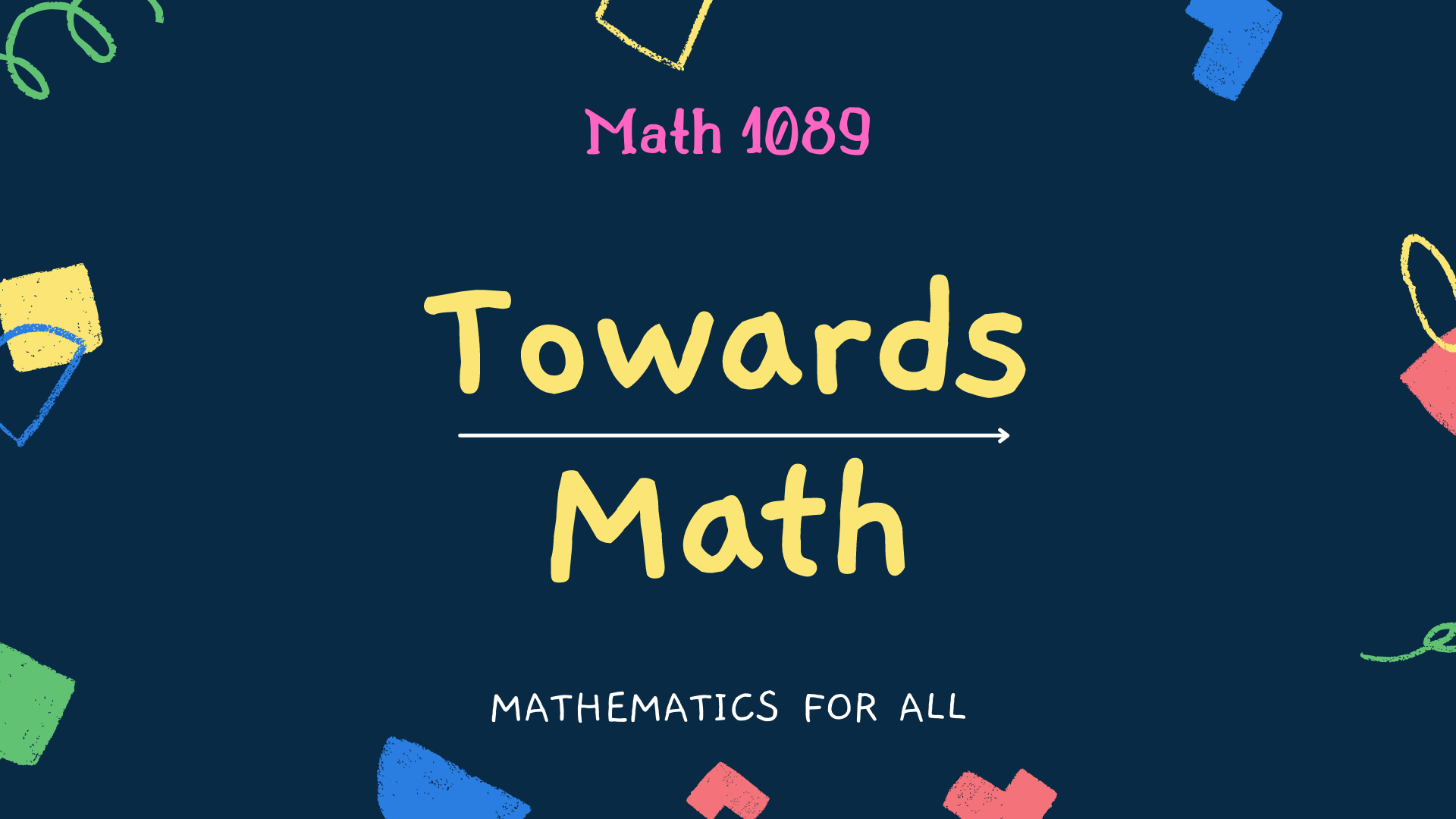# Towards Math

𝗜𝗻 𝘁𝗵𝗶𝘀 𝗰𝗶𝗿𝗰𝗹𝗲, 𝗮𝗹𝗹 𝘁𝗵𝗲 𝗻𝘂𝗺𝗯𝗲𝗿𝘀 𝗳𝗿𝗼𝗺 𝟭 𝘁𝗼 𝟭𝟬𝟬 𝗮𝗿𝗲 𝘁𝗵𝗲𝗿𝗲, 𝘄𝗶𝘁𝗵𝗼𝘂𝘁 𝗿𝗲𝗽𝗲𝘁𝗶𝘁𝗶𝗼𝗻. 𝗧𝗵𝗲 𝘀𝘂𝗺 𝗼𝗳 𝗮𝗻𝘆 𝘁𝘄𝗼 𝗰𝗼𝗻𝘀𝗲𝗰𝘂𝘁𝗶𝘃𝗲 𝗶𝗻𝘁𝗲𝗴𝗲𝗿𝘀 𝗶𝘀 𝗮 𝗽𝗲𝗿𝗳𝗲𝗰𝘁 𝘀𝗾𝘂𝗮𝗿𝗲.

For example,

19 + 17 = 36 = 62

16 + 20 = 36 = 62

25 + 39 = 64 = 82

37 + 27 = 64 = 82

56 + 25 = 81 = 92

39 + 42 = 81 = 92

44 + 56 = 100 = 102

18 + 82 = 100 = 102

58 + 63 = 121 = 112

57 + 64 = 121 = 112

82 + 62 = 144 = 122

100 + 44 = 144 = 122

80 + 89 = 169 = 132

1.Sandeep kumar says:

Very interesting sir.

1.Math1089 says:

Thank you Sir

2.StayCalm says:

37 itself is interesting, and so are 37037, 37037037, etc. for similar reasons.

1.Math1089 says:

These are in pipeline. Thank you for your suggestion.

2.souravkumarbejgmailcom says:

Wow…like this we can create more square numbers!!

1.Math1089 says:

Thank you Sourav.

3.Alex Marshall says:

Wow! Next time around, it’s Math for me!

1.Math1089 says:

Good to hear this. Thank you so much.

4.Carey Wrye says:

1.Math1089 says: